# 从头开始复现YOLOv3（二）数据类

wuchangjian2021-10-31 17:15:57编程学习

### 创建数据类

• 1 数据组织
• （1）coco128数据集
• （2）从训练集中划分出验证集
• 2 为数据集创建类

# 1 数据组织

## （1）coco128数据集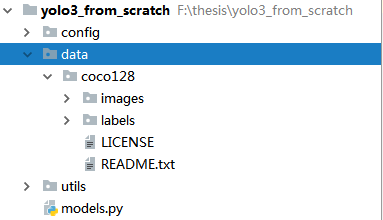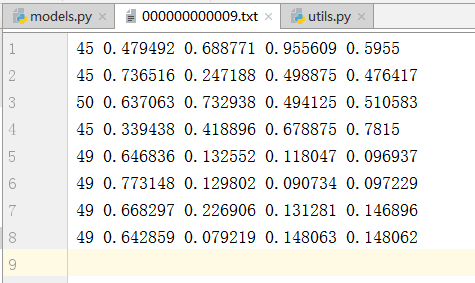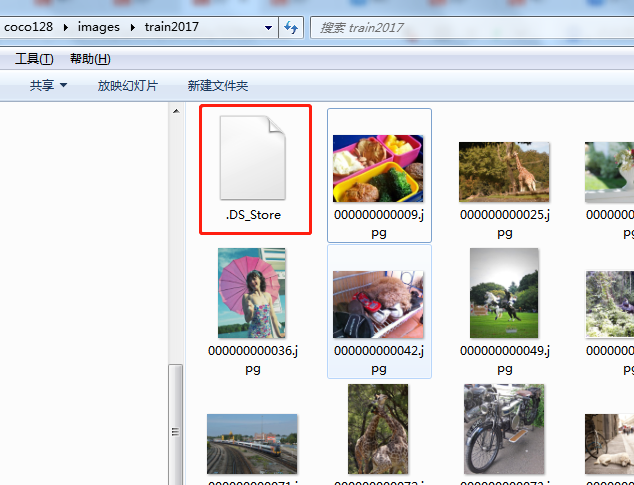## （2）从训练集中划分出验证集

``````import os
import random
random.seed(10)

# 从图片路径中获取图片名
images_path = r"F:\thesis\yolo3_from_scratch\data\coco128\images\train2017"
images_names = os.listdir(images_path)      # 以列表的方式返回一级子目录
images_num = len(images_names)

# 随机获取10个数，作为图片名列表的索引，进而获得图片名
num_val = 10                # 验证集的数目
idx_img = random.sample(range(0, images_num), num_val)   # 生成不重复的num_val个随机数

# 生成验证集样本的名字，构成一个列表
val_names = [images_names[i] for i in idx_img]
# print(val_names)

# 生成训练集样本的名字，构成一个列表
train_names = [images_names[i] for i in range(images_num) if i not in idx_img]
# print(train_names)

# 将训练集样本的路径写入txt文件
with open(r"F:\thesis\yolo3_from_scratch\data\coco128\train_path.txt", 'w') as f:
for file_name in train_names:
f.write(os.path.join(images_path, file_name)+'\n')

# 将验证集样本的路径写入txt文件
with open(r"F:\thesis\yolo3_from_scratch\data\coco128\val_path.txt", 'w') as f:
for file_name in val_names:
f.write(os.path.join(images_path, file_name)+'\n')
``````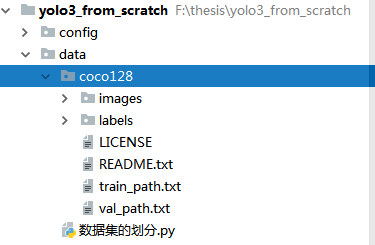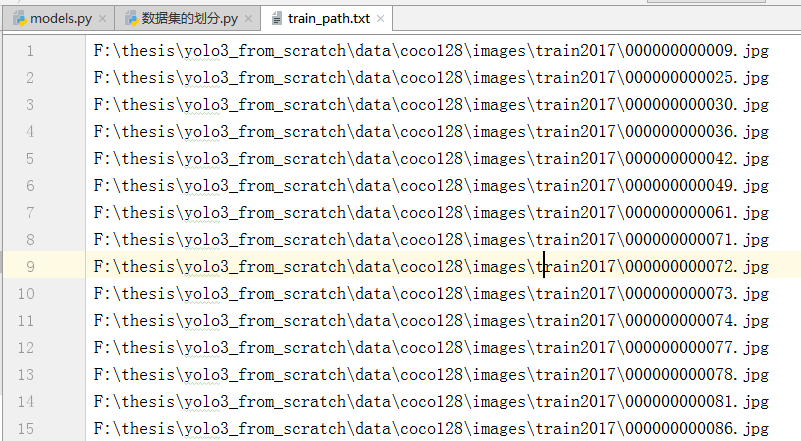val_path.txt文件内容如下：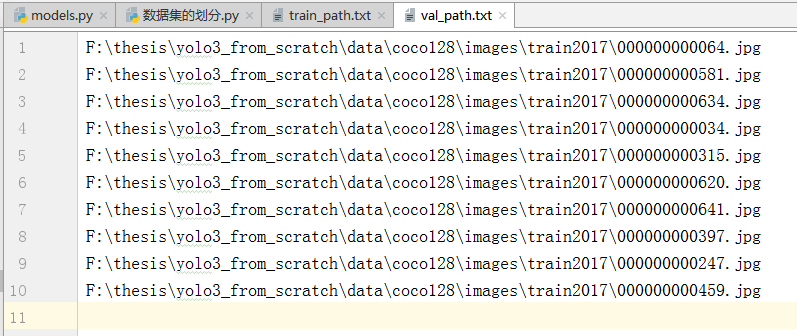# 2 为数据集创建类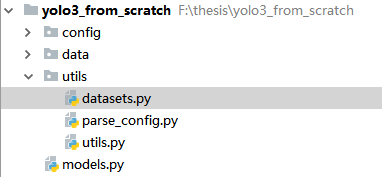``````import os
from PIL import Image
import torch
from torch.utils.data import Dataset
import torchvision.transforms as transforms
import numpy as np
import random
random.seed(0)
``````

``````class ListDataset(Dataset):
def __init__(self, list_path, img_size=416, augment=True, multiscale=True, normalized_labels=True):
'''

:param list_path: 一个txt文件，比如我们前面写的train_path.txt和val_path.txt
:param img_size: 数据图片要转成成的高
:param augment: 是否使用数据增强
:param multiscale: 是否进行多尺度变换（看self.collate_fn就能明白它的作用）
:param normalized_labels: 标签是否已经归一化，即boundingbox的中心坐标，高宽等是否已经归一化
'''
with open(list_path, "r") as file:

# 标签的路径，可以根据样本的路径来获得，只需要将路径名中的images改成labels，后缀改成txt就行
self.label_files = [
path.replace("images", "labels").replace(".png", ".txt").replace(".jpg", ".txt")
for path in self.img_files
]
self.img_size = img_size    # 图片处理成方形后的高宽（图片在输入模型前要处理成方形）
self.max_objects = 100      # 一张图片中的最大目标数
self.augment = augment
self.multiscale = multiscale
self.normalized_labels = normalized_labels
self.min_size = self.img_size - 3 * 32  # 在进行多尺度变换时的最小尺度
self.max_size = self.img_size + 3 * 32  # 在进行多尺度变换时的最大尺度
self.batch_count = 0                    # 统计已经遍历了多少个batch
# TODO max_objects是用来干嘛的
``````

``````    def __len__(self):
return len(self.img_files)
``````

``````    def __getitem__(self, index):
img_path = self.img_files[index % len(self.img_files)].rstrip()     # 获取图片的路径名

# Extract image as PyTorch tensor
img = transforms.ToTensor()(Image.open(img_path).convert('RGB'))    # 读取图片并转化为torch张量
# mage.open(img_path)读取图片，返回Image对象，不是普通的数组
# convert('RGB')进行通道转换，因为当图像格式为RGBA时，Image.open(‘xxx.jpg’)读取的格式为RGBA

# Handle images with less than three channels
if len(img.shape) != 3:
img = img.unsqueeze(0)
img = img.expand((3, img.shape[1:]))
# 图片有可能是一张灰度图，那么img.shape就是（h, w）
# unsqueeze(0)之后，就是img.shape就是（1, h, w）
# img.expand((3, img.shape[1:])) 即为 img.expand((3, h, w))

_, h, w = img.shape
h_factor, w_factor = (h, w) if self.normalized_labels else (1, 1)
# h_factor, w_factor在后面用来反算目标在图片中的具体坐标，看到本函数的后面，自然能明白
# 如果已经归一化，那么比例因子就是图片的真实高宽
# 如果未归一化，那比例因子就是1

``````

``````import torch.nn.functional as F
"""
该函数是将图片扩充成正方形
:param img: 图片张量
:return:
"""
c, h, w = img.shape
dim_diff = np.abs(h - w)

# 如果h小于w，那么就是在上下填充，否则在左右填充

``````

``````        label_path = self.label_files[index % len(self.img_files)].rstrip() # 获取标签路径

targets = None
if os.path.exists(label_path):
f = open(label_path, 'r')
# 有些图片没有目标，但有标签文件，这些标签文件中没有内容
# 我们这边只处理有内容的标签文件，对于没有内容的标签文件，让targets等于None

# Extract coordinates for unpadded + unscaled image
# 获取bbox左上角和右下角点在原始图片上的真实坐标
x1 = w_factor * (boxes[:, 1] - boxes[:, 3] / 2)
y1 = h_factor * (boxes[:, 2] - boxes[:, 4] / 2)
x2 = w_factor * (boxes[:, 1] + boxes[:, 3] / 2)
y2 = h_factor * (boxes[:, 2] + boxes[:, 4] / 2)

# Returns (x, y, w, h)
# 求归一化后的中心点坐标和高宽
boxes[:, 1] = ((x1 + x2) / 2) / padded_w
boxes[:, 2] = ((y1 + y2) / 2) / padded_h
boxes[:, 3] *= w_factor / padded_w
boxes[:, 4] *= h_factor / padded_h

targets = torch.zeros((len(boxes), 6))
targets[:, 1:] = boxes          # 后面5列分别是bbox的位置和高宽，然后是分类索引
# target第0列，根据后面的collate_fn函数，可以看到第0列是图片在batch中的索引

# Apply augmentations
# 随机进行水平翻转
if self.augment:
if np.random.random() < 0.5:
img, targets = horisontal_flip(img, targets)

f.close()

return img_path, img, targets

``````

``````import torch
def horisontal_flip(images, targets):
"""
水平翻转
:param images:图片张量
:param targets:标签
:return:
"""
images = torch.flip(images, [-1])   # 按照指定维度进行翻转，-1表示最后一个维度，即为宽
targets[:, 2] = 1 - targets[:, 2]   # 中心点横坐标也要随机翻转，因为已经归一化，所以直接用1减就行
return images, targets
``````

``````from utils.augmentations import horisontal_flip
``````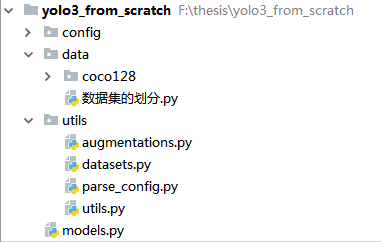``````# coding=utf-8
import torch
from utils.datasets import ListDataset

train_path = r"F:\thesis\yolo3_from_scratch\data\coco128\train_path.txt"
dataset = ListDataset(train_path, augment=True, multiscale=True)

dataset,
batch_size=2,
shuffle=True
)
``````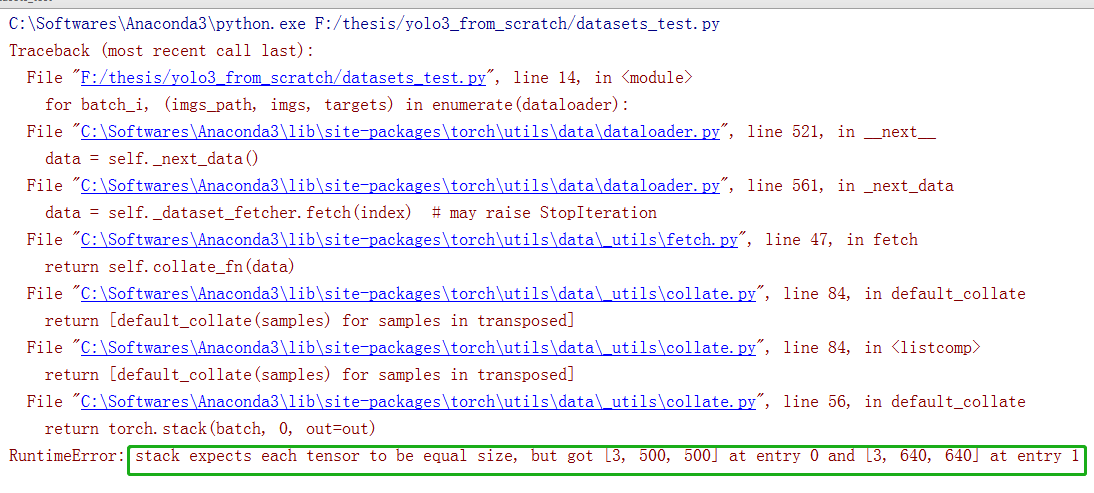https://blog.csdn.net/qq_43391414/article/details/120462055

``````    def collate_fn(self, batch):
"""
用于整理数据
:param batch: 若干次__getitem__函数返回的内容组成的列表
假如说batch_size为2，那么batch就是由2个元素组成的列表，
每个元素代表一次__getitem__函数的返回结果
__getitem__函数的返回值有三个部分组成：img_path, img, targets
那么batch的每个元素就是一个元组，包含了img_path, img, targets
:return:
"""
paths, imgs, targets = list(zip(*batch))    # zip括号中的参数*开头，表示解压缩
# 上条命令执行之后，paths, imgs, targets都将成为元组，
# 以paths为例，上述命令执行后，paths将成为由两个图片路径构成的元组

# Add sample index to targets 将图片在batch中的索引，加到target的第0个列
for i, boxes in enumerate(targets):
if boxes is not None:
boxes[:, 0] = i     # i表示当前batch中的第i张图片

# Remove empty placeholder targets 有些图片没有目标，那么它对应的标签就是None
targets = [boxes for boxes in targets if boxes is not None]  # 保留非None的标签

targets = torch.cat(targets, 0)     # 标签级联，targets在转化前是一个元组

# Selects new image size every tenth batch
if self.multiscale and self.batch_count % 10 == 0:  # 每10个batch，随机改变一下尺度
self.img_size = random.choice(range(self.min_size, self.max_size + 1, 32))
# range函数的第三个参数是32，能保证随机获得的新尺寸是32的倍数，
# 因为是backbone是32倍下采样，如果不是32的倍数，那么卷积核不能完全把图片扫描

# 将图片缩放到指定尺寸
imgs = torch.stack([resize(img, self.img_size) for img in imgs])    #
self.batch_count += 1

# 图片缩放之后，之所以标签不用改变，是因为标签已经归一化了，所以无需转换

return paths, imgs, targets
``````

``````# 这段代码与YOLOv3无关，仅仅解释list(zip(*batch))实现了什么
a = ('a', 25, 1)
b = ('b', 43, 0)
L= [a, b]
m = zip(*L)     # zip对象
print(m)
print(list(m))

``````

``````<zip object at 0x00000000026828C0>
[('a', 'b'), (25, 43), (1, 0)]
``````

collate_fn中出现了resize函数，可以在datasets.py中加上这个函数：

``````def resize(image, size):
"""
将图片缩放成指定尺寸
:param image: 图片张量
:param size:  指定尺寸，高和宽都是这个值，也就是说，本函数缩放的是正方形
:return:
"""
image = F.interpolate(image.unsqueeze(0), size=size, mode="nearest").squeeze(0)
return image
``````

``````# coding=utf-8
import torch
from utils.datasets import ListDataset

train_path = r"F:\thesis\PyTorch-YOLOv3\data\coco\trainvalno5k.txt"
dataset = ListDataset(train_path, augment=True, multiscale=True)

dataset,
batch_size=2,
shuffle=True,
collate_fn=dataset.collate_fn,  #
)

for batch_i, (_, imgs, targets) in enumerate(dataloader):
print(batch_i)
print("imgs.shape:", imgs.shape)
print("targets.shape:", targets.shape)
break

``````

``````0
imgs.shape: torch.Size([2, 3, 384, 384])
targets.shape: torch.Size([6, 6])
``````

``````# 测试batch为5的时候，第18个batch的targets中，是否有图片索引为1的行
# 1表示第2张图片，即000000000508.jpg的在batch中的索引，而000000000508.jpg中没有目标
# 因为000000000508.jpg就在train_path.txt的第87行，因为这张图片的索引为86，是在第18个batch中
# 可以看看返回的targets的最后几行的图片索引

import torch
from utils.datasets import ListDataset

train_path = r"F:\thesis\yolo3_from_scratch\data\coco128\train_path.txt"
dataset = ListDataset(train_path, augment=True, multiscale=True)
dataset,
batch_size=5,
shuffle=False,              # 这边不能打乱
collate_fn=dataset.collate_fn,  #
)

for batch_i, (imgs_path, imgs, targets) in enumerate(dataloader):
if batch_i<17:
continue

print(targets[:3, :])   # 只显示前三行，因为索引为85的图片，只有一个目标
# 如果第2行以2开头，那说明程序修改的正确，如果以1开头，说明程序出问题
break

# 通过输出可以看到，targets的第2行是以2开头，说明程序对00....0508.txt处理得当。
``````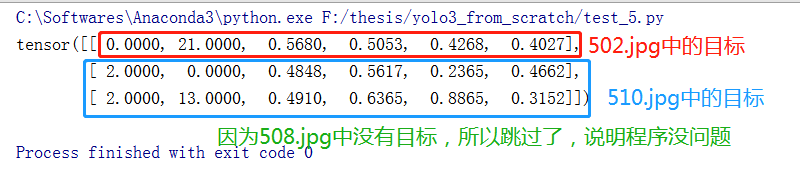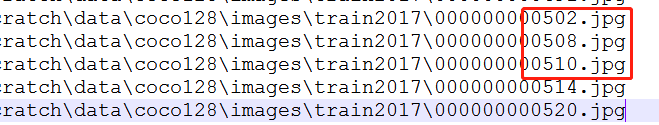...

...

### SpringCloud 和Dubbo 的区别

Dubbo关注的领域是Spring  Cloud的一个子集。Dubbo专注与服务治理&#...

### 2021年中国高校大数据挑战赛A题完整版

...

#### 发表评论◎欢迎参与讨论，请在这里发表您的看法、交流您的观点。